# JP5259761B2 - Data conversion apparatus and program - Google Patents

## Info

Publication number
JP5259761B2
JP5259761B2 JP2011063230A JP2011063230A JP5259761B2 JP 5259761 B2 JP5259761 B2 JP 5259761B2 JP 2011063230 A JP2011063230 A JP 2011063230A JP 2011063230 A JP2011063230 A JP 2011063230A JP 5259761 B2 JP5259761 B2 JP 5259761B2
Authority
JP
Japan
Prior art keywords
unit
power usage
array
power
server
Prior art date
Legal status (The legal status is an assumption and is not a legal conclusion. Google has not performed a legal analysis and makes no representation as to the accuracy of the status listed.)
Expired - Fee Related
Application number
JP2011063230A
Other languages
Japanese (ja)
Other versions
JP2012200094A (en
Inventor

Original Assignee

Priority date (The priority date is an assumption and is not a legal conclusion. Google has not performed a legal analysis and makes no representation as to the accuracy of the date listed.)
Filing date
Publication date
Application filed by 株式会社東芝 filed Critical 株式会社東芝
Priority to JP2011063230A priority Critical patent/JP5259761B2/en
Publication of JP2012200094A publication Critical patent/JP2012200094A/en
Application granted granted Critical
Publication of JP5259761B2 publication Critical patent/JP5259761B2/en
Application status is Expired - Fee Related legal-status Critical
Anticipated expiration legal-status Critical

• 238000006243 chemical reaction Methods 0 abstract 1
• 238000003860 storage Methods 0 abstract 1

## Images

•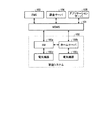•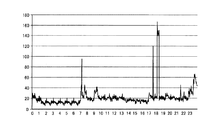•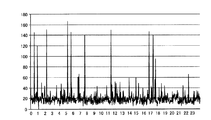•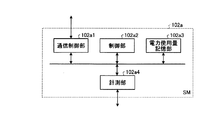•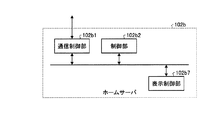•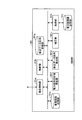•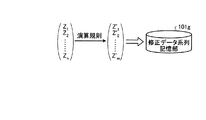•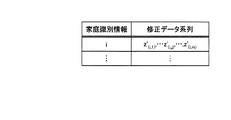•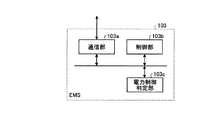•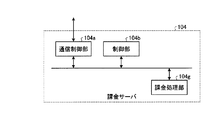•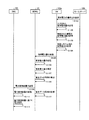•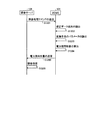•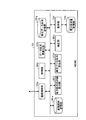•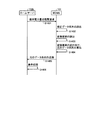•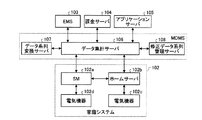•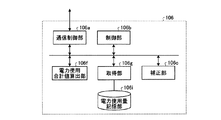•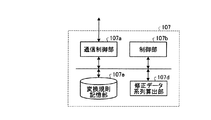•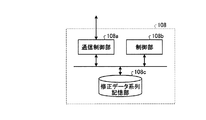•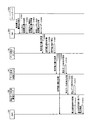•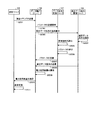•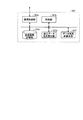•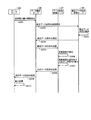## Classifications

• GPHYSICS
• G01MEASURING; TESTING
• G01RMEASURING ELECTRIC VARIABLES; MEASURING MAGNETIC VARIABLES
• G01R22/00Arrangements for measuring time integral of electric power or current, e.g. by electricity meters
• G01R22/06Arrangements for measuring time integral of electric power or current, e.g. by electricity meters by electronic methods
• G01R22/10Arrangements for measuring time integral of electric power or current, e.g. by electricity meters by electronic methods using digital techniques
• GPHYSICS
• G06COMPUTING; CALCULATING; COUNTING
• G06QDATA PROCESSING SYSTEMS OR METHODS, SPECIALLY ADAPTED FOR ADMINISTRATIVE, COMMERCIAL, FINANCIAL, MANAGERIAL, SUPERVISORY OR FORECASTING PURPOSES; SYSTEMS OR METHODS SPECIALLY ADAPTED FOR ADMINISTRATIVE, COMMERCIAL, FINANCIAL, MANAGERIAL, SUPERVISORY OR FORECASTING PURPOSES, NOT OTHERWISE PROVIDED FOR
• G06Q30/00Commerce, e.g. shopping or e-commerce
• G06Q30/04Billing or invoicing, e.g. tax processing in connection with a sale

## Abstract

According to an embodiment, a data converting device is connected to a power meter configured to add up power consumptions of electrical equipment. The device includes a receiving unit configured to receive power consumptions per predetermined unit time added up by the power meter; and a calculating unit configured to calculate a second sequence to be stored as added up power consumptions in a storage unit from a first sequence containing the power consumptions per unit time received by the receiving unit in time series by using a conversion rule for converting a value of a second element in the second sequence based on a value of a first element in the first sequence. The second sequence has no privacy information and, the second sequence can be used for calculating a total value of the first sequence.

## Description

Embodiments of the present invention, the data conversion apparatus relates及 beauty program.

In addition to conventional power generation such as nuclear power and thermal power, power supply tends to diversify by using renewable energy such as sunlight and wind power. In order to stabilize the quality of power when diversifying this power supply, the construction of a next-generation power network (smart grid) has been proposed. In the next-generation power network, power management will be performed by installing smart meters (hereinafter also referred to as SMs) that aggregate power consumption and home servers that manage electrical devices in each home or business location. There is a need. A meter data management system (hereinafter also referred to as MDMS) and an energy management system (hereinafter also referred to as EMS) connected to a smart meter via a next-generation power network are connected to each home and each It manages the power used at the office and controls the power supply to each home and each office.

For example, a smart meter communicates with a meter data management system and transmits the amount of power used at home or business. The meter data management system stores and manages the power consumption transmitted from the smart meter at each home or office at regular time intervals. The energy management system suppresses the use of power to the smart meter and home server of each home or office based on the power consumption of multiple homes or offices stored in the meter data management system. It performs power control such as requesting or controlling charging / discharging of a storage battery connected to the next generation power network.

Furthermore, it has been proposed to provide various services using the power consumption managed by the meter data management system. Providing these services can be realized by connecting an application server loaded with various applications to the next-generation power network. An example of such an application server is a billing server managed by a provider. Such a billing server performs billing processing based on the amount of power used by each home or office managed by the meter data management system.

Furthermore, the power consumption amount managed by the meter data management system can be transmitted to the smart meter upon request. For example, when the meter data management system receives a power usage amount browsing request from a smart meter, information such as the power usage amount managed by the meter data management system can be disclosed.

JP 2004-112868 A

However, when the power consumption of each home or office is managed by the storage device provided in the meter data management system, the meter data management system administrator or an unauthorized The three parties will be able to view the power consumption of each household and business establishment. In this way, when an administrator or an unauthorized third party views the amount of power used, it is possible to infer whether the home or business is at home, the state of activity, and the like, which causes a problem of privacy infringement.

The data conversion device according to the embodiment is a data conversion device connected to a power meter that aggregates the power usage of an electric device, and includes a reception unit, a calculation unit, and a total value calculation unit . Receiver, the power meter was counted, receives power usage per unit predetermined time. The calculation unit is a conversion rule that converts the value of the second element based on the value of an arbitrary first element of the array, and can restore each element of the array before conversion from the array after conversion using the conversion rule, from the first sequence stored in the chronological order of power consumption per unit receiving unit receives time as elements, calculating a second sequence to be stored in the storage unit as the power consumption obtained by aggregating To do. Sum calculation unit, by calculation technique based on the conversion rule, from the sum of the elements of the second array, without restoring the respective elements of the first array, calculating the sum of the elements of the first array To do.

FIG. 1 is a diagram illustrating a configuration of a power usage amount calculation system according to the first embodiment. FIG. 2 is a diagram exemplifying power usage amounts collected by the power usage amount calculation system according to the first embodiment. FIG. 3 is a diagram illustrating a modified data series managed by the power usage amount calculation system according to the first embodiment. FIG. 4 is a diagram illustrating a configuration of SM functions according to the first embodiment. FIG. 5 is a diagram illustrating a functional configuration of the home server according to the first embodiment. FIG. 6 is a diagram illustrating a functional configuration of MDMS according to the first embodiment. FIG. 7 is a diagram illustrating an operation of the correction data series calculation unit according to the first embodiment. FIG. 8 is a diagram illustrating a table structure included in the modified data sequence storage unit according to the first embodiment. FIG. 9 is a diagram illustrating a configuration of functions of the EMS according to the first embodiment. FIG. 10 is a diagram illustrating a functional configuration of a billing server according to the first embodiment. FIG. 11 is a sequence diagram illustrating a procedure of total power consumption calculation processing according to the first embodiment. FIG. 12 is a sequence diagram illustrating a procedure of a billing system process according to the first embodiment. FIG. 13 is a diagram illustrating a functional configuration of MDMS according to the first modification of the first embodiment. FIG. 14 is a sequence diagram illustrating a procedure of browsing request processing according to the first modification of the first embodiment. FIG. 15 is a diagram illustrating a configuration of a power usage amount calculation system according to the second embodiment. FIG. 16 is a diagram illustrating a functional configuration of a data totaling server according to the second embodiment. FIG. 17 is a diagram illustrating a functional configuration of a data series conversion server according to the second embodiment. FIG. 18 is a diagram illustrating a functional configuration of a modified data sequence management server according to the second embodiment. FIG. 19 is a sequence diagram illustrating a procedure of total power consumption calculation processing according to the second embodiment. FIG. 20 is a sequence diagram illustrating a procedure of a billing system process according to the second embodiment. FIG. 21 is a diagram illustrating a functional configuration of the data series conversion server according to the first modification of the second embodiment. FIG. 22 is a sequence diagram illustrating a procedure of browsing request processing according to the first modification of the second embodiment.

(First embodiment)
FIG. 1 is a block diagram illustrating a configuration of a power usage amount calculation system according to the first embodiment. In the example of the power usage calculation system shown in FIG. 1, a meter data management system (MDMS) 101, a home system 102, an energy management system (EMS) 103, and a billing server 104 are connected via a network or the like. ing. For simplification of the drawing, only one home system 102 is shown, but a plurality of home systems 102 can be connected to the power usage amount calculation system. Examples of the network include a LAN (Local Area Network), an intranet, Ethernet (registered trademark), and the Internet.

The MDMS 101 is a system that collects and manages the power consumption of each home via a network or the like.

The home system 102 is a system that counts the amount of electric power used by electric devices installed in the home and used at home. The smart meter (SM) 102a, the home server 102b, the electric device 102c, and the electric device 102d. And have. The electric device 102c is connected to the home server 102b by wire or wirelessly. The home server 102b manages the power usage of the subordinate electric device 102c and controls the subordinate electric device 102c. The electric device 102d is connected to the SM 102a by wire or wirelessly. The SM 102a receives the power usage of the electrical device 102c via the home server 102b, and totals the power usage in the home system 102 together with the power usage of the electrical device 102d. For simplification of the figure, an example is shown in which one electrical device is connected to each of the smart meter 102a and the home server 102b. However, in the smart meter 102a and the home server 102b, the power consumption in the home is actually Various electrical devices are connected to measure.

First, the outline | summary of the electric power consumption calculation system concerning this embodiment is demonstrated. As shown in FIG. 1, in the power usage amount calculation system, an MDMS 101 is connected to an SM 102a installed for each home system. The MDMS 101 manages the power usage per predetermined unit time as an array for each home or each office. FIG. 2 is a diagram showing the power consumption of each home or business in time series. As shown in FIG. 2, when the power consumption is shown in time series, there is a risk that it will be understood whether there are people in each household or each office or what kind of electrical equipment is provided. is there.

Therefore, the meter data management system (MDMS) 101 according to the present embodiment is arranged in an array of power consumption per unit time (hereinafter referred to as a data series) indicated in time series order of each home or each office. On the other hand, using a predetermined conversion rule that makes each element of the array different, an array (hereinafter referred to as a corrected data series) that preserves the power consumption of each home or each office and protects privacy is calculated. The corrected data series is stored. FIG. 3 is a diagram showing an example in which the corrected data series calculated from the data series shown in FIG. 2 is graphed. As shown in FIG. 3, in the modified data series generated according to a predetermined conversion rule, it is difficult to grasp the power usage amount of each home or each office, so that privacy can be protected. In other words, by managing as a correction data series, even when the correction data series is illegally browsed, it is possible to grasp the time to use the electrical equipment and electrical equipment provided in each home or office. Can be suppressed. Furthermore, based on such information, it is possible to prevent identification of the preference or behavior of the individual or group, or identification of the individual or group itself.

However, when the MDMS 101 stores the amount of electricity used per unit time as a modified data series, if there is a request from another application (for example, the billing server 104) or the like, the amount of power used by each home or office Need to send the exact sum of Therefore, the conversion rule used by the MDMS 101 in the present embodiment is that the total value of power usage per unit time (hereinafter referred to as “reset data sequence”) is not returned from the corrected data sequence to the original power usage consumption per unit time sequence. , Also referred to as the total amount of electricity used). As a result, when the MDMS 101 calculates the total power usage in response to a request from an application or the like, it is not necessary to return the corrected data sequence to the original time-sequential arrangement of the power usage per unit time. It is possible to prevent leakage of privacy information and improve safety.

In this way, the MDMS 101 outputs the total power usage amount derived from the corrected data series in response to a request from the billing server 104 or the like. Note that the information that the MDMS 101 derives from the corrected data series without returning to the original time-sequential arrangement of the power usage per unit time is not limited to the total power usage, but is requested by the application or the like. Any information may be used.

In order to identify the home system 102, identification information (referred to as home identification information) is assigned to each home system. The home server 102b and the SM 102a store home identification information assigned to the home system 102. Each of the MDMS 101, the EMS 103, and the billing server 104 stores the home identification information of all the home systems 102 connected to the power usage amount calculation system.

Then, the MDMS 101 receives from the SM 102a the power usage amount in the first unit time for each home system counted by the SM 102a. The first unit time is an appropriate unit time for the MDMS 101 to manage the power consumption of each home or office, and may be a time interval for the EMS 103 to control the power network. In this embodiment, 15 minutes is taken as an example.

Then, the MDMS 101 calculates and stores a corrected data series based on a predetermined conversion rule from the data series in which the received power usage amounts in the first unit time are arranged in time series. Note that the power usage amount received by the MDMS 101 from the SM 102a is associated with the home identification information. Further, the information received from the SM 102a by the MDMS 101 is not limited to the information in which the power usage amount in the first unit time is associated with the home identification information, but may be associated with other information. .

Then, the MDMS 101 calculates information according to the purpose of the application without restoring the data series in which the power usage amounts in the original first unit time are arranged in time series order from the stored corrected data series. . The calculated information is, for example, information related to the power usage amount of each home or office used by the application, such as the total power usage amount in an individual home or office.

In addition, the correction data series used for calculation by the MDMS 101 may be a plurality of correction data series based on the power usage amounts respectively tabulated by different SMs 102a. The application requesting the provision of information to the MDMS 101 is, for example, a charging processing application realized by the charging server 104 or various applications realized by the other application server 105.

As described above, the MDMS 101 transmits the calculated information to each application server 105. Thereafter, the application server 105 performs processing by the activated application using the received information.

Next, the hardware configuration of the MDMS 101, SM 102a, home server 102b, EMS 103, and accounting server 104 will be described. In this embodiment, the accounting server 104, the EMS 103, and the MDMS 101 are examples of servers.

The MDMS 101, the EMS 103, and the billing server 104 according to the present embodiment include a control unit such as a CPU (Central Processing Unit) that executes control of the entire apparatus and basic operations, and a ROM (Read Only Memory) that stores various data and various programs. A main storage unit such as a RAM (Random Access Memory), a storage unit such as an HDD (Hard Disk Drive) that stores various data and various programs, an auxiliary storage unit such as a CD (Compact Disk) drive device, etc. And a hardware configuration using a normal computer. Further, the MDMS 101 and the EMS 103 include a communication I / F (Interface) that performs communication via a network or the like.

The SM 102a and the home server 102b are a control unit such as a CPU (Central Processing Unit) that controls the entire apparatus, a ROM (Read Only Memory) that stores various data and various programs, and a main memory such as a RAM (Random Access Memory). And an auxiliary storage unit such as a nonvolatile memory for storing various data and various programs, and a bus for connecting them, and has a configuration similar to that of dedicated hardware or embedded equipment. Furthermore, the SM 102a and the home server 102b include a communication I / F (Interface) that communicates with an external device via a network or the like. In addition, the home server 102b is connected to a display unit that displays various types of information such as power consumption, and an operation input unit such as an operation button or a keyboard to which a user operation is input.

Next, various functions implemented in each of the MDMS 101, SM 102a, home server 102b, EMS 103, and billing server 104 in such a hardware configuration will be described.

First, various functions realized by the SM 102a will be described. FIG. 4 is a diagram illustrating a functional configuration of the SM 102a. As shown in FIG. 4, the SM 102a includes a communication control unit 102a1, a control unit 102a2, a power usage amount storage unit 102a3, and a measurement unit 102a4. These configurations include the execution of various programs stored in the main storage unit and the auxiliary storage unit by the CPU of the SM 102a, the use of the communication I / F (not shown) of the SM 102a, and the auxiliary storage unit of the SM 102a. This is realized by a storage area secured in the memory.

The communication control unit 102a1 controls communication with other devices such as the home server 102b and the MDMS 101.

The control unit 102a2 controls the SM 102a as a whole.

The measuring unit 102a4 aggregates the power usage amount z (i, j) of the electric devices 102c and 102d for each first unit time. The variable i is an index for identifying the home system 102. The variable j is an index corresponding to the first unit time order (date, time, etc.).

Then, the measurement unit 102a4 stores the total power usage amount in the power usage amount storage unit 102a3. At this time, the measurement unit 102a4 performs the device authentication for the electric device 102d via the communication control unit 102a1, and then calculates the power usage amount used by the electric device 102d at least once in the first unit time. The data is stored in the storage unit 102a3. At the same time, the measurement unit 102a4 stores the power usage amount used by the electric device 102c managed by the home server 102b described later in the power usage amount storage unit 102a3. In this embodiment, the power usage amounts of the electric devices 102c and 120d are totaled for each first unit time as described above, but the totaling method is not limited.

The power usage amount storage unit 102a3 stores the power usage amount counted by the measurement unit 102a4.

Next, various functions implemented in the home server 102b will be described. FIG. 5 is a diagram illustrating a functional configuration of the home server 102b. As shown in FIG. 5, the home server 102b includes a communication control unit 102b1, a control unit 102b2, and a display control unit 102b7. In these configurations, the CPU of the home server 102b executes various programs stored in the main storage unit and the auxiliary storage unit, uses the communication I / F (not shown) of the home server 102b, and further the home server 102b. This is realized by a display unit (not shown) or the like.

The communication control unit 102b1 controls communication with other devices such as the SM 102a and the MDMS 101. In communication with other devices, encryption communication such as TLS / SSL or SIP (Session Initiation Protocol) may be performed.

The control unit 102b2 controls the entire home server 102b. In addition, when the power usage amount of the subordinate electric device 102c is written in the SM 102a, the control unit 102b2 measures the power usage amount of the electric device 102c at least once in the first unit time, and passes the communication control unit 102b1. Then, the SM 102a is accessed, and the measured power usage is stored in the SM 102a.

In addition, the control unit 102b2 controls display processing that accompanies a request for browsing the power consumption. For example, the control unit 102b2 displays the power usage amount when an operation input unit (not shown) that requests browsing of the power usage amount is received. In the display process, first, the control unit 102b2 generates a browsing request command for requesting browsing of power usage. Then, the communication control unit 102b1 transmits the generated browsing request command to the MDMS 101. It should be noted that the period during which the power usage amount is desired to be browsed (referred to as the desired browse period) may be determined in advance or may be specified by a user operation input via the operation input unit. The desired browsing period and home identification information are included in the browse request command generated by the control unit 102b2.

Then, the communication control unit 102b1 receives, from the MDMS 101, the power usage amount z (i, j) in one or more first unit times corresponding to the desired browsing period included in the browsing request command. Then, in accordance with control by the control unit 102b2, the display control unit 102b7 displays the received power usage amount power usage amount z (i, j) on the display unit. In addition, although the display part connected to the home server 102b was used for the display of the electric power consumption in a browsing process, you may utilize the output terminal (not shown) connected to a home system.

Next, various functions implemented in the MDMS 101 will be described. FIG. 6 is a diagram illustrating a functional configuration of the MDMS 101. As shown in FIG. 6, the MDMS 101 includes a communication control unit 101a, a control unit 101b, a correction unit 101c, a modified data series calculation unit 101d, a conversion rule storage unit 101e, and a power usage total value calculation unit 101f. A correction data series storage unit 101g, an acquisition unit 101i, and a power usage amount storage unit 101j. These configurations are based on the communication I / F of the MDMS 101, the execution of various programs stored in the main storage unit and the auxiliary storage unit by the CPU of the MDMS 101, and the storage area secured in the storage unit such as the HDD of the MDMS 101. Realized.

The control unit 101b controls the entire MDMS 101.

The communication control unit 101a controls communication with other devices such as the SM 102a, the EMS 103, the billing server 104, and the application server 105. In communication with other devices, encryption communication such as TLS / SSL or SIP (Session Initiation Protocol) may be performed. For example, the communication control unit 101a accesses the SM 102a every first unit time, and receives the power usage amount z (i, j) stored in the power usage amount storage unit 102a3 of the SM 102a from the SM 102a. As another example, the communication control unit 101a receives a browsing request command from the home server 102b, or transmits the power usage amount z (i, j) to the home server 102b in response to the browsing request command. Furthermore, the communication control unit 101a transmits to the EMS 103 the total power usage for each first unit time of a plurality of homes calculated by the control unit 101b. Furthermore, the communication control unit 101a receives a charging process command for instructing execution of the charging system process from the charging server 104. In response to the charging processing command, the communication control unit 101a transmits to the charging server 104 the total power usage amount in each home for the second unit time calculated by the power usage total value calculation unit 101f.

The acquisition unit 101i acquires the power usage amount used in each home system 102 including various electrical devices for each first unit time received from the SM 102a via the communication control unit 101a.

The power usage amount storage unit 101j stores a plurality of power usage amounts for each first unit time acquired by the acquisition unit 101i from the SM 102a.

Correction unit 101c examines whether there is an error in the power usage is stored in the power consumption storage unit 101j z (i, j), correction for optionally power usage z (i, j) The amount is calculated, and the power usage amount z (i, j) is corrected using the correction amount. For example, the acquiring unit 101i acquires the power usage amount for each first unit time from the same SM 102a, and then stores it in the power usage amount storage unit 101j. Then, the correction unit 101c checks whether or not there is an error in the power usage amount z (i, j) stored in the power usage amount storage unit 101j, and if an error is detected, the power usage amount z (i , j) is corrected and stored in the power consumption storage unit 101j again. The power consumption after correction is also indicated as z (i, j) .

In addition, the control unit 101b calculates the power usage amount z (i, j) stored in the power usage amount storage unit 101j after the correction unit 101c corrects the plurality of home systems 102 for each first unit time. Then, the total power usage a (j) = Σ i z (i, j) of a plurality of households for each first unit time ( j) is calculated.

The corrected data series calculation unit 101d uses a predetermined conversion rule (A, b) to indicate a data series z (i, i, i) indicating the power usage amount for each first unit time stored in the power usage amount storage unit 101j . From j) , the corrected data sequence z ′ (i, j) stored in the corrected data sequence storage unit 101g as the total power consumption is calculated. The predetermined conversion rule (A, b) is a value of an arbitrary element included in the power usage amount z (i, j) indicating the power usage amount for each first unit time in time series for each home identification information. Based on a conversion rule that changes the values of other elements based on the calculated data series , the total value of the original power consumption z (i, j) before the calculation can be calculated from the modified data sequence generated by the calculation based on the conversion rule And

FIG. 7 is an explanatory diagram showing the calculation of the corrected data series calculation unit 101d. As illustrated in FIG. 7, the corrected data sequence calculation unit 101d calculates a corrected data sequence z ′ (i, j) from the data sequence z (i, j) using a predetermined conversion rule (A, b). And stored in the corrected data series storage unit 101g.

The corrected data series calculation unit 101d may calculate the corrected data series when all the elements of the data series including the power usage amount z (i, j) of each household are complete, or the power usage of each household. The corrected data sequence may be calculated each time a partial element of the data sequence composed of the quantity z (i, j) (or every time the power usage amount z (i, j) is received) is prepared.

The correction data series storage unit 101g stores the correction data series calculated by the correction data series calculation unit 101d. FIG. 8 is a diagram illustrating an example of a table structure provided in the modified data sequence storage unit 101g. As shown in FIG. 8, the corrected data series storage unit 101g stores a corrected data series in association with each home identification information.

A data series (z (i, 1) , z (i, 2) ,..., Z (i, n ) composed of power consumptions in n first unit times for each home system 102 (home identification information i). ) ) Is the original data series. The modified data sequence calculation unit 101d according to the present embodiment uses a predetermined conversion rule (A, b) to generate a data sequence (z (i, 1) , z (i, 2) , ..., z (i, n). ) ) To calculate the corrected data series (z ′ (i, 1) , z ′ (i, 2) ,..., Z ′ (i, m) ) and set in advance in the corrected data series storage unit 101g for each element. Stored data (S [i, 1], S [i, 2], ..., S [i, m]) are stored in the modified data series (z ′ (i, 1) , z ′ (i, 2) , ..., z ' (i, m) ) is memorized. In this way, by setting the storage area for each element (S [i, 1], S [i, 2],..., S [i, m] in advance, every time a part of the data series is prepared, the correction data A series can be calculated.

The predetermined conversion rule (A, b) used by the corrected data sequence calculation unit 101d for calculating the corrected data sequence will be described. The predetermined conversion rule (A, b) according to the present embodiment is composed of a matrix A having m rows and n columns and a column vector b having m rows. Then, the corrected data series calculation unit 101d calculates the corrected data series using the following equation (1).

(z ' (i, 1) , z' (i, 2) , ..., z ' (i, m) ) T = A (z (i, 1) , z (i, 2) , ..., z (i , n) ) T + b (1)

The conversion rule (A, b) will be described. The matrix A of the conversion rules (A, b) is set so that the sum of the elements in each column is c [A] in all columns. The sum of all elements of the column vector b of the conversion rule (A, b) is c [b]. This conversion rule (A, b) is set by the SM 102a or the MDMS 101. The superscript T represents transposition. For example, (z ′ (1) , z ′ (2) ,..., Z ′ (n) ) T represents a column vector composed of n rows.

As described above, the correction data series may be calculated sequentially, or after a plurality or all of z (i, j) have been prepared. In the case of performing sequentially, first, storage areas (S , S [] for storing (z ′ (i.1) , z ′ (i, 2) ,..., Z ′ (i, n) )) 2], ..., S [m]) is initialized with (0,0, ..., 0). Each time the acquisition unit 101i acquires z (i, j) , the corrected data series calculation unit 101d performs the j-th column A (.: J) and z (i ) of A as shown in Expression (2). , j) is calculated and added to (S , S ,..., S [m]).

(S , S , ..., S [m]) T = (S , z , ..., S [m]) T + A (・: j) * z (i , j) (2)

Then, after the addition using all z (i, j) is completed, the modified data sequence calculation unit 101d converts each element of the column vector b into (S , S ,..., S [ m]).

The conversion rule storage unit 101e stores the conversion rule (A, b) used in the modified data series calculation unit 101d.

The power usage total value calculation unit 101f is a second unit based on a calculation method predetermined as corresponding to the conversion rule (A, b) for the correction data sequence stored in the correction data sequence storage unit 101g. A total value of power used in the home system 102 (total amount of power used) is calculated by summing up each element of the original data series for each hour. The total power usage value calculation unit 101f according to the present embodiment calculates the total power usage amount used in the second unit time in response to a request from the accounting server 104. The second unit time is a unit of time for which the charging server 104 performs the charging process, and is set to one month, for example. Thus, the second unit time is set as including a plurality of first unit times.

In this embodiment, an example of concealing the amount of power used in each home will be described. However, it is only necessary to conceal the amount of power used in the aggregation range (aggregation unit) of the smart meter that uses power. It does not limit the amount of electricity used by each household.

By the way, the total sum Σz (i, j) (total power usage ) of the elements of the data series for each household system 102 and the total sum Σz ′ ( The following equation (3) is established between i, j) . Note that c [A] is the sum of the elements of each column of the matrix A, and c [b] is the sum of the elements of the column vector b.

That is, the sum Σz (i, j) (total power consumption ) of the elements of the data series can be derived by the following equation (4).

Thus, the power usage total value calculation unit 101f uses c [A] and c [b] when obtaining the sum Σz (i, j) (total power usage ) of the elements of the data series from the corrected data series. Thus, the total power consumption can be calculated. In other words, in this embodiment, c [A] and c [b] are used in the calculation method corresponding to the conversion rule (A, b).

For example, when m = n = 3, c [A] = 1, and c [b] = 0, the corrected data series calculation unit 101d uses the matrix A and the column vector b shown below to change the corrected data series. calculate.

In this case, for example, the corrected data series calculation unit 101d calculates the corrected data series (5, 2, 6) from the data series (4, 2, 7). Then, the power usage total value calculation unit 101f calculates the total power usage amount from Expression (5).
(Σz ′ (i, j) −c [b]) / c [A] = ((5 + 2 + 6) −0) / 1 = 13 (5)

It can be confirmed that 13 calculated from this equation (5) matches Σd [i] = 4 + 2 + 7 = 13.

In the case of m <n, for example, when m = 2, n = 3, c [A] = 2, and c [b] = 1, the corrected data series is used using the matrix A and the column vector b shown below. The calculation unit 101d calculates a corrected data series.

In this case, for example, the corrected data series calculation unit 101d calculates the corrected data series (-8, 35) from the data series (4, 2, 7). Then, the power usage total value calculation unit 101f calculates the total power usage amount from Expression (6).
(Σz ′ (i, j) −c [b]) / c [A] = ((− 8 + 35) −1) / 2 = 13 (6)

It can be confirmed that 13 calculated from the equation (6) matches Σd [i] = 4 + 2 + 7 = 13.

Further, the same applies when m> n. For example, when m = 4, n = 3, c [A] = 3, and c [b] = − 1, the following matrix A and column vector b are obtained. Using, the correction data series calculation unit 101d calculates the correction data series.

In this case, for example, the corrected data series calculation unit 101d calculates the corrected data series (35, 3, -5, 5) from the data series (4, 2, 7). Then, the power usage total value calculation unit 101f calculates the total power usage amount from Expression (7).
(Σz ′ (i) −c [b]) / c [A] = ((35 + 3 + (− 5) +5-(− 1)) / 3 = 13 (7)

It can be confirmed that 13 calculated from this equation (7) matches Σd [i] = 4 + 2 + 7 = 13.

In the MDMS 101 according to the present embodiment, the power consumption of each household can be concealed by storing the power consumption of each household as a modified data series in the modified data series storage unit 101g. it can.

Next, various functions realized in the EMS 103 will be described. The EMS 103 performs power control using the sum of the power usage amounts of a plurality of households for each first unit time.

FIG. 9 is a diagram illustrating a functional configuration of the EMS 103. As illustrated in FIG. 9, the EMS 103 includes a communication unit 103a, a control unit 103b, and a power control determination unit 103c. These configurations are realized by the communication I / F of the EMS 103 and the CPU of the EMS 103 executing various programs stored in the main storage unit and the auxiliary storage unit.

The control unit 103b controls the entire EMS 103.

The communication unit 103a controls communication with other devices such as the MDMS 101. In communication with other devices, encryption communication such as TLS / SSL or SIP (Session Initiation Protocol) may be performed. The communication unit 103 a receives the total power usage amount a (j) for each first unit time (j) from the MDMS 101. In addition, the communication unit 103a transmits a power control command for controlling use of power to the SM 102a and the home server 102b under the control of the control unit 103b.

The power control determination unit 103c determines whether or not to perform power control based on the total power usage amount in the first unit time received by the communication unit 103a. With power control, for example, when the total power usage exceeds the upper threshold, the use of power in each home is suppressed, and when the total power usage is below the lower threshold, the storage battery is charged. I will do it. The power control determination unit 103c generates a power control command for requesting suppression of power use when it is determined to perform control for suppressing power use in each home. Then, the communication unit 103a transmits the generated power control command to the SM 102a and the home server 102b.

Next, various functions implemented in the accounting server 104 will be described. FIG. 10 is a diagram illustrating a functional configuration of the accounting server 104. As shown in FIG. 10, the accounting server 104 includes a communication control unit 104a, a control unit 104b, and an accounting processing unit 104g. These configurations are realized by the communication I / F included in the accounting server 104 and the CPU included in the accounting server 104 executing various programs stored in the main storage unit and the auxiliary storage unit.

The control unit 104b controls the charging server 104 as a whole.

The communication control unit 104a controls communication with other devices such as the MDMS 101. In communication with other devices, encryption communication such as TLS / SSL or SIP (Session Initiation Protocol) may be performed. According to the control of the control unit 104b, the communication control unit 104a transmits a charging process command for instructing execution of the charging system process to the MDMS 101 every second unit time. In addition, the communication control unit 104 a receives the total power usage amount of each home for each second unit time from the MDMS 101.

The billing processing unit 104g performs billing processing based on the second total power usage amount of each home system 102 for each second unit time received by the communication control unit 104a.

Next, a procedure of processing performed by the power usage amount calculation system according to the present embodiment will be described. FIG. 11 is a sequence diagram showing the procedure of the total power usage calculation process in the power usage calculation system. In the example shown in FIG. 11, the MDMS 101 generates a conversion rule (A, b) in advance and stores it in the conversion rule storage unit 101e. The conversion rule (A, b) may be different for each home, or may be different for each second unit time. When the conversion rule (A, b) is different for each home or every second unit time, the conversion rule storage unit 101e of the MDMS 101 indicates the conversion rule (A, b), the home identification information and the second unit time. The information is stored in association with the information. The MDMS 101 is not limited to generating the conversion rule (A, b), and the conversion rule (A, b) may be received from another device and stored in the conversion rule storage unit 101e.

The home server 102b makes a write request to the SM 102a at least once every first unit time for the amount of power used by the connected electrical device 102c (step S1101). As a result, the SM 102a stores the input power usage amount of the electric device 102c in an auxiliary storage area (not shown). (Step S1102). Further, the SM 102a stores the power usage amount of the electric device 102d in an auxiliary storage area (not shown) at least once every first unit time in accordance with a request from the electric device 102d (step S1103). Note that the control of step S1102 and step S1103 may be performed spontaneously by the SM 102a.

Further, the SM 102a totals the written power usage amounts of the electric devices 102c and 102d for each first unit time, and stores them in the power usage amount storage unit 102a3 (step S1104).

The communication control unit 101a of the MDMS 101 accesses the SM 102a at least once in the first unit time, and receives the power usage amount z (i, j) stored in the power usage amount storage unit 102a3 (step S1105). At that time, the communication control unit 101a also receives home identification information given to the home system 102 from the SM 102a.

The control unit 101b stores the received power usage amount z (i, j) in the power usage amount storage unit 101j (step S1106). At that time, the home identification information is also stored in association with the power usage amount z (i, j) .

When the correcting unit 101c checks whether there is an error in the power usage amount z (i, j) for each home system 102 stored in the power usage amount storage unit 101j, A correction amount is calculated, and the power usage amount z (i, j) is corrected using the correction amount (step S1107). Then, the power usage amount z (i, j) corrected by the correction unit 101c is stored again in the power usage amount storage unit 101j. The MDMS 101 may transmit the corrected value to the SM 102a.

For example, the correction unit 101c of the MDMS 101 detects and corrects an error in the power usage amount z (i, j) using the power usage amount z (i, j ′) for a plurality of j ′ in the same home system 102. To do. Note that the correction unit 101c uses the power usage amount z (i, j ′) that satisfies “j ′> j” in order to perform error detection and correction of the power usage amount z (i, j). Also good. For example, the correcting unit 101c uses the power consumption amount z (i, j-1) of the first unit time “j-1” received from the SM 102a and the first unit time “j + 1” received from the SM 102a. Using the power usage amount z (i, j + 1 ) , error detection and correction of the power usage amount z (i, j) in the first unit time j are performed in the following procedure.

The communication control unit 101a of the MDMS 101 receives z (i, j-1) , z (i, j) , and z (i, j + 1) from the SM 102a every time the first unit time elapses. Thereafter, the correction unit 101c of the MDMS 101 performs error detection using a predetermined threshold th. For example, the correcting unit 101c includes the received power usage amount z (i, j) in the range of “((z (i, j−1) + z (i, j + 1) ) / 2) ± th”. It is determined whether or not. Then, the correction unit 101c regards the power usage amount z (i, j) as “(z (i, j− 1) Correct to + z (i, j + 1) ) / 2 ”.

In the case of using a power usage z (i, j) "j in order to perform error detection and correction of 'power usage becomes>j" z (i, j'), the processing after step S1108 is, SM 102 Alternatively, the power usage amount z (i, j ′) may be read out from the data, and an error detection and correction of the power usage amount z (i, j) may be performed by the correction unit 101c. It should be noted that some processes that require immediacy such as power control by the EMS 103 may be performed without performing error detection and correction of the power usage amount z (i, j) .

The corrected data sequence calculation unit 101d of the MDMS 101 uses the corrected data sequence based on the power consumption amount z (i, j) of the home system 102 and the conversion rule (A, b) stored in the conversion rule storage unit 101e. Is calculated (step S1108). As described above, the timing of calculating the corrected data series is not limited. For example, the correction data series is corrected after receiving a part of the data series (the power usage amount in one or more first unit times). A data series may be calculated. When the conversion rule (A, b) is different for each home or every second unit time, the corrected data series calculation unit 101d converts the conversion rule (A, b) associated with the home identification information or the second unit time. Use b).

Further, the control unit 101b of the MDMS 101 totals the power usage amounts z (i, j) of the plurality of home systems 102, and the total power usage amount “a (j) of the plurality of homes for each first unit time (j)”. = Σ i z (i, j) ”is calculated, and the total power consumption a (j) of a plurality of households for each first unit time is transmitted to the EMS 103 via the communication control unit 101a (step S1109). Note that the processing shown in steps S1106 to S1109 above may be executed in any order.

Then, the correction data sequence calculation unit 101d of the MDMS 101 stores the calculated correction data sequence in the correction data sequence storage unit 101g (step S1110). If the power usage amount z (i, j) is not used for error detection and correction and calculation (update) of the corrected data series after the storage is completed, the control unit 101b of the MDMS 101 determines that the power usage amount z (i, j) may be deleted from the RAM (main storage unit) temporarily stored as a work area.

The communication unit 103a of the EMS 103 receives, from the MDMS 101, the total power consumption amount a (j) of a plurality of households counted for each first unit time and stores it in, for example, the main storage unit (Step S1111. Total power usage amount a). (j) may be received from the MDMS 101 every time the first unit time elapses, or a plurality of corresponding power usage totals may be received after the plurality of first unit times elapses.

Thereafter, the EMS 103 performs power control based on the total power usage amount a (j) for each first unit time (step S1112). After step S1112, the EMS 103 may delete the total power usage amount a (j) for each first unit time from the main storage unit.

Next, the charging system processing procedure performed by the power usage amount calculation system will be described. When the power consumption total amount calculation process shown in FIG. 11 is executed, a modified data sequence storing the consumed power amount for each first unit time is stored in the modified data sequence storage unit 101g. Whenever the second unit time elapses, the billing server 104 receives the total amount of power used in the second unit time of each household from the MDMS 101 and performs billing processing. FIG. 12 is a sequence diagram illustrating a procedure of a charging system process including a charging process in the power usage amount calculation system.

First, the billing server 104 transmits a billing process command for instructing execution of the billing system process to the MDMS 101 every second unit time (step S1201). In the charging process command, the second unit time to be charged and the home identification information are designated. The charging process command may be transmitted from the MDMS 101 to the charging server 104 instead of from the charging server 104.

When the communication control unit 101a of the MDMS 101 receives the billing processing command, the power usage total value calculation unit 101f calculates the power usage amount correction data series (z ′ () corresponding to the specified home identification information and the second unit time. i, 1) , z ′ (i, 2) ,..., z ′ (i, m) ) are read from the modified data sequence storage unit 101g (step S1202). Furthermore, the power usage total value calculation unit 101f reads the sum c [A], which is a parameter of the conversion method corresponding to the conversion rule (A, b), and the sum c [b] of the elements of b (step S1203). ).

The total power usage value calculation unit 101f of the MDMS 101 uses the total c [A], the total c [b], and the corrected data series, and uses the second unit time in Expression (8) shown below. The calculated total power consumption is calculated (restored) (step S1204).

Σ j z (i, j) = (Σ (j ′) z ′ (i, j ′) − c [b]) / c [A] (8)

The index i corresponds to home identification information. The index j corresponds to each element of the data series in the second unit time, and takes a value from 1 to n. The index j 'corresponds to each element of the modified data series in the second unit time and takes a value from 1 to m.

Then, the communication control unit 101a of the MDMS 101 transmits the calculated total power usage amount Σ j z (i, j) of the second unit time to the accounting server 104 (step S1205). The MDMS 101 transmits the total power consumption Σ j z (i, j) to the billing server 104 and, after a predetermined time has elapsed, the corrected data series (z ′ (i, 1) , z ′ (i, j, 2) ,..., Z ′ (i, m) ) may be deleted from the modified data sequence storage unit 101g. Here, the predetermined time is a period during which there is a possibility that retransmission of the second power usage total amount is accepted from the accounting server 104, and is, for example, three months.

The billing server 104 performs billing processing corresponding to the amount of power used in the second unit time for each home based on the received total power usage (step S1206).

As described above, in the MDMS 101 of the present embodiment, the power usage amount in each first unit time for each home is converted into a corrected data sequence using the conversion rule (A, b), and then the corrected data sequence is stored. Stored in the unit 101g. Then, simply referring to the corrected data sequence stored in the corrected data sequence storage unit 101g cannot restore the power consumption of each household for each first unit time. That is, even if the correction data series leaks due to erroneous processing by the administrator of the MDMS 101, incorrect access control settings, unauthorized intrusion, etc., it is possible to suppress leakage of the power usage amount for each first unit time for each home. . For this reason, it is impossible to infer whether the third party is at home according to the time or the state of the activity from the leaked corrected data series, and the privacy of each home can be protected.

In addition, the EMS 103 according to the present embodiment performs power control using the total power consumption in a plurality of homes in the first unit time as an application server. Therefore, in the present embodiment, the MDMS 101 calculates the total amount of power usage in the first unit time in a plurality of homes from the power usage in the first unit time for each home, and the calculation result is sent to the EMS 103. Send. As a result, the EMS 103 can grasp the total power usage amount in the first unit time in a plurality of homes, but cannot grasp the power usage amount in each first unit time for each home, thus protecting the privacy of each home. be able to.

Further, in the present embodiment, an example has been described in which the billing server 104 that performs billing processing with the total power consumption for each home in the second unit time is used as the application server. In the present embodiment, the MDMS 101 calculates the total power usage amount for each second unit time for each home from the power usage amount for each first unit time for each home, and transmits the calculation result to the billing server 104. Yes. As a result, the billing server 104 can grasp the total power usage per second unit time for each home, but cannot grasp the power usage per first unit time for each home, thus protecting the privacy of each home. it can.

(Modification 1 of the first embodiment)
In the first embodiment, the matrix A used for the conversion rule may be any matrix as long as the sum of the elements in each column is c [A]. On the other hand, the matrix A used as the conversion rule in the first modification of the first embodiment will be described in the case where the sum of the elements of each column is c [A] and is a regular matrix.

FIG. 13 is a diagram illustrating a functional configuration of the MDMS 101 according to the first modification of the first embodiment. Compared to the MDMS 101 of the first embodiment, the MDMS 101 according to the first modification of the first embodiment shown in FIG. 13 has a data series recalculation unit 101h added. Note that other systems and functional configurations of the power consumption calculation system according to the present modification are the same as those in the first embodiment, and thus description thereof is omitted.

The data series recalculation unit 101h uses the inverse matrix of the regular matrix A included in the conversion rule (A, b) stored in the conversion rule storage unit 101e for the corrected data series to convert the original data series. calculate. Thus, in this modification, since the matrix A included in the conversion rule (A, b) is a regular matrix, an inverse matrix can be derived. In other words, by using the inverse matrix, the corrected data can be derived. The original data series can be calculated from the series.

As described above, the MDMS 101 according to the first modification of the first embodiment, in response to a power usage amount browsing request from a home, shows the original data series indicating the power usage per unit time in the home. Can be sent.

The conversion rule (A, b) stored in the conversion rule storage unit 101e is stored in an area that cannot be referenced from the outside. Alternatively, the conversion rule storage unit 101e stores the encrypted conversion rule (A, b). This prevents an unauthorized third party from browsing the conversion rule (A, b) and calculating the original data series.

Note that the total power consumption calculation process and the billing process are the same as the procedure shown in the first embodiment, and a description thereof will be omitted. Then, in the charging system process shown in FIG. 12 of the first embodiment, the MDMS 101 corrects the corrected data sequence after a predetermined time (a period during which the retransmission of the total power usage amount may be received from the charging server 104). It may be deleted from the modified data series storage unit 101g. On the other hand, in this modification, in order to reply to the browsing request process from the SM 102a, there is a possibility that the retransmission of the total power usage amount may be received from the accounting server 104, or the browsing request process may be received from the home system 102. The corrected data series is stored for a certain period. The storage period is, for example, 10 years.

Next, a procedure of browsing request processing performed by the power usage amount calculation system according to this modification will be described. Note that, before the browsing request process is performed, a total power consumption calculation process is performed, and a corrected data series (z ′ (i, 1) , z ) composed of the power consumption for each first unit time of each household. It is assumed that ' (i, 2) , ..., z' (i, n) ) is stored in the modified data sequence storage unit 101g in association with the home identification information. FIG. 14 is a sequence diagram illustrating a procedure of browsing request processing in the power usage amount calculation system.

The home server 102b of the home system 102 transmits a browsing request command Req_i for requesting browsing of power consumption to the MDMS 101 (step S1401).

When the communication control unit 101a of the MDMS 101 receives the browsing request command Req_i, the data series recalculation unit 101h is stored in association with the home identification information and the browsing request period specified by the browsing request command Req_i. The data series (z ′ (i, 1) , z ′ (i, 2) ,..., Z ′ (i, m) ) is read (step S1402). Further, the data series recalculation unit 101h reads the conversion rule (A, b) from the conversion rule storage unit 101e (step S1403). When the browse request period extends over a plurality of second unit times, a plurality of corresponding correction data series and conversion rules are read out.

The data sequence recalculation unit 101h of the MDMS 101 uses an inverse matrix of the normal matrix A included in the conversion rule (A, b) or the like, from the modified data sequence, the original data sequence (in which the power usage is shown in chronological order) z (i, 1) , z (i, 2) ,..., z (i, n) ) are calculated (step S1404). Equation (9) used for the calculation is shown below.

(z (i, 1) , z (i, 2) , ..., z (i, n) ) = A -1 ((z ' (i, 1) , z' (i, 2) , ..., z ' (i, m) ) -b) (9)
Here, A −1 is an inverse matrix of A. A -1 may be calculated in advance when A is generated and may be stored together with A in the conversion rule storage unit 101e, or may be calculated from the normal matrix A in response to a browsing request.

Then, the communication control unit 101a of the MDMS 101 uses z (i, k) , z (i, k + 1) ,..., Z (i, l) corresponding to the browsing request period in the calculated original data series. And transmitted to the home server 102b (step S1405). Note that 1 <k <l <n.

The home server 102b displays z (i, k) , z (i, k + 1) ,..., Z (i, l) received from the MDMS 101 on the display unit (step S1406). Thereby, the browsing request process ends.

In the modified example of the first embodiment, since the conversion rule is stored so that it cannot be referred to, the first unit time in each household can be obtained by simply referring to the corrected data series as in the first embodiment. It is not possible to calculate the amount of power used every time. Thereby, the privacy of each home can be protected. Similarly to the first embodiment, the privacy of each home can be protected for the EMS 103 and the billing server 104 in the modified example.

(Modification 2 of the first embodiment)
Further, in the above-described embodiment and the modification, the example using the (conversion) matrix as the conversion rule has been described. However, the conversion rule is not limited to using a matrix.

Therefore, in the second modification of the first embodiment, a case where conversion using a matrix is not performed will be described. The modified data sequence calculation unit 101d of this modification changes the order of the data sequences z (i, 1) , z (i, 2) , ..., z (i, n) according to a predetermined rule. The modified data series z ′ (i, 1) , z ′ (i, 2) ,..., Z ′ (i, n) are generated. The corrected data series z ′ (i, 1) , z ′ (i, 2) ,..., Z ′ (i, n) generated in this way are calculated by the power usage total value calculation unit 101f of the corrected data series. By summing up each element, the total value of the data series can be calculated.

In this way, even in the modified data series generated by shuffling each element of the data series, the third party cannot recognize the power usage amount according to the time series of each household, so privacy can be protected. it can. In addition, since it is easy to calculate the correction data series and calculate the total power consumption, the processing load of the MDMS 101 can be reduced. Note that the shuffling of each element of such a data series can also be understood as a calculation based on a regular matrix in which the matrix A of the conversion rule (A, b) has one “1” in each row and column.

(Second Embodiment)
In the first embodiment, the case where MDMS is one server has been described. However, it is not limited that MDMS is a single server. Therefore, in the second embodiment, an example in which a plurality of servers is applied to the MDMS of the power usage calculation system will be described. In addition, about the part which is common in the above-mentioned 1st Embodiment, description is abbreviate | omitted using the same code | symbol.

Therefore, in the power usage amount calculation system according to the second embodiment, the calculation of the correction data series and the storage of the correction data series are executed by different servers.

FIG. 15 is a diagram illustrating a configuration of a power usage amount calculation system according to the second embodiment. As shown in FIG. 15, the power usage calculation system includes a meter data management system (MDMS) including a data totaling server 106, a data series conversion server 107, and a modified data series management server 108, a home system 102, An energy management system (EMS) 103 and an accounting server 104 are provided, and these are connected via a network or the like. For simplification of the drawing, only one home system 102 is shown, but a plurality of home systems 102 can be connected to the power usage amount calculation system.

The data totaling server 106 collects the power usage amount of each home via a network or the like, calculates the total power usage amount (total value of power usage amount) as necessary, and transmits it to other servers and the like. For example, the data totaling server 106 calculates the total power usage amount of the plurality of homes for each first unit time based on the power usage amount of the plurality of homes, and transmits it to the EMS 103. As another example, the data totaling server 106 calculates the total power usage amount of each home for each second unit time from the corrected data series and transmits it to the accounting server 104. Then, when calculating the total power usage amount of each home for each second unit time from the corrected data sequence, the data tabulation server 106 restores the data sequence consisting of the original power usage amount for each first unit time. Even if it is possible to calculate the total power consumption of each household, it is possible to prevent leakage of privacy information.

The data series conversion server 107 calculates a corrected data series based on the power usage amount for each first unit time of each household and the conversion rule (A, b) described above. The calculation method is the same as the method described in the first embodiment, and a description thereof is omitted.

The correction data series management server 108 stores and manages the correction data series calculated by the data series conversion server 107.

Next, the hardware configuration of the data totaling server 106, the data series conversion server 107, and the modified data series management server 108 will be described. The data totaling server 106, the data series conversion server 107, and the modified data series management server 108 are a control unit such as a CPU (Central Processing Unit) that executes control of the entire apparatus and basic operations, and various data and various programs. Main storage unit such as ROM (Read Only Memory) and RAM (Random Access Memory) for storage, storage unit such as HDD (Hard Disk Drive) for storing various data and various programs, and CD (Compact Disk) drive device And the like, a communication I / F (Interface) that performs communication via a network, and a bus that connects these, a hardware configuration using a normal computer.

Next, various functions implemented in each of the data totaling server 106, the data series conversion server 107, and the modified data series management server 108 in such a hardware configuration will be described.

First, various functions implemented in the data totaling server 106 will be described. FIG. 16 is a diagram illustrating a functional configuration of the data totaling server 106. As shown in FIG. 16, the data totaling server 106 includes a communication control unit 106a, a control unit 106b, a correction unit 106c, a power usage total value calculation unit 106f, an acquisition unit 106g, and a power usage amount storage unit 106i. . In these configurations, the CPU of the data totaling server 106 executes various programs stored in the main storage unit and the auxiliary storage unit, the use of the communication I / F (not shown) of the data totaling server 106, and the data totaling server This is realized by a storage area secured in a storage unit such as an HDD of 106.

The control unit 106b controls the entire data totaling server 106.

The communication control unit 106a controls communication with other devices such as the SM 102a, the EMS 103, the billing server 104, and the application server 105. In communication with other devices, encryption communication such as TLS / SSL or SIP (Session Initiation Protocol) may be performed. For example, the communication control unit 106a accesses the SM 102a every first unit time, and stores the power usage amount z (i, j per first unit time) stored in the power usage amount storage unit 102a3 of the SM 102a. ) From the SM 102a. As another example, the communication control unit 101a receives a browsing request command from the home server 102b, or transmits the power usage amount z (i, j) to the home server 102b in response to the browsing request command. Furthermore, the communication control unit 106a transmits to the EMS 103 the total power usage for each first unit time of the plurality of homes calculated by the control unit 106b. Furthermore, the communication control unit 101a receives a charging process command for instructing execution of the charging system process from the charging server 104. In response to the charging processing command, the communication control unit 101a transmits to the charging server 104 the total power usage amount in each home for each second unit time calculated by the total power usage value calculation unit 101f.

The acquisition unit 106g acquires the power usage amount used in each home system 102 including various electric devices for each first unit time received from the SM 102a via the communication control unit 106a.

The power usage amount storage unit 106i stores a plurality of power usage amounts for each first unit time acquired by the acquisition unit 106g from the SM 102a.

Correction unit 106c examines whether there is an error in the power usage is stored in the power usage amount storing unit 106i z (i, j), correction for optionally power usage z (i, j) The amount is calculated, and the power usage amount z (i, j) is corrected using the correction amount. The specific correction method is the same as that in the first embodiment.

In addition, the control unit 106b calculates the power usage amount z (i, j) stored in the power usage amount storage unit 106i after the correction unit 106c corrects the plurality of home systems 102 for each first unit time. Then, the total power consumption a (j) = Σ i z (i, j) of a plurality of households for each first unit time is calculated.

The total power use value calculation unit 106f is predetermined for the correction data sequence received from the correction data sequence management server 108 as corresponding to the conversion rule (A, b) used by the data sequence conversion server 107 for conversion. The total value of power used by the home system 102 (total amount of power used) is calculated by summing up the elements of the original data series every second unit time by the calculation method.

Next, various functions implemented in the data series conversion server 107 will be described. FIG. 17 is a diagram illustrating a functional configuration of the data series conversion server 107. As shown in FIG. 17, the data series conversion server 107 includes a communication control unit 107a, a control unit 107b, and a modified data series calculation unit 107d. These configurations are realized by the communication I / F of the data series conversion server 107 and the CPU of the MDMS 101 executing various programs stored in the main storage unit and the auxiliary storage unit.

The communication control unit 107a controls communication with other devices such as the data totaling server 106. In communication with other devices, encryption communication such as TLS / SSL or SIP (Session Initiation Protocol) may be performed.

The conversion rule storage unit 107e stores the conversion rule (A, b) used in the modified data series calculation unit 107d.

The corrected data series calculation unit 107d uses the conversion rule (A, b) described above, and the data series z (i, i) indicates the power usage amount of each household received by the communication control unit 107a for each first unit time . From j) , a corrected data series is calculated. As described above, the corrected data series calculation unit 107d may calculate the corrected data series when all the data series z (i, j) indicating the power usage amount of each household are prepared, or the power usage of each household. The corrected data sequence may be calculated after a part of the data sequence z (i, j) indicating the amount (or every time the power usage amount z (i, j) is received) is prepared.

Next, various functions implemented in the modified data sequence management server 108 will be described. FIG. 18 is a diagram illustrating a functional configuration of the correction data series management server 108. As shown in FIG. 18, the correction data sequence management server 108 includes a communication control unit 108a, a control unit 108b, and a correction data sequence storage unit 108c. These configurations include the communication I / F of the correction data sequence management server 108, the execution of various programs stored in the main storage unit and the auxiliary storage unit by the CPU of the correction data sequence management server 108, and the correction data sequence management server 108. This is realized by a storage area secured in a storage unit such as an HDD.

The control unit 108b controls the entire modified data series management server 108.

The communication control unit 108a controls communication with other devices such as the data totaling server 106. In communication with other devices, encryption communication such as TLS / SSL or SIP (Session Initiation Protocol) may be performed.

When the communication control unit 108a receives the correction data sequence calculated by the data sequence conversion server 107, the correction data sequence storage unit 108c stores the correction data sequence in association with the home identification information.

Next, a procedure of processing performed by the power usage amount calculation system according to the present embodiment will be described. First, the procedure for calculating the total power consumption will be described with reference to FIG. In the example shown in FIG. 19, the data series conversion server 107 generates a conversion rule (A, b) in advance and stores it in the conversion rule storage unit 101e. The conversion rule (A, b) may be different for each home, or may be different for each second unit time. When the conversion rule (A, b) is different for each home or every second unit time, the conversion rule storage unit 101e of the MDMS 101 indicates the conversion rule (A, b), the home identification information and the second unit time. The information is stored in association with the information. The MDMS 101 is not limited to generating the conversion rule (A, b), and the conversion rule (A, b) may be received from another device and stored in the conversion rule storage unit 101e.

Then, similarly to steps S1101 to S1104 in FIG. 11 of the first embodiment, the SM 102a aggregates the power usage amount of the home system 102 for each first unit time and stores it in the power usage amount storage unit 102a3. (Steps S1901 to S1904).

The communication control unit 106a of the data totaling server 106 accesses the SM 102a at least once in the first unit time, and receives the power usage amount z (i, j) stored in the power usage amount storage unit 102a3 (step S1905). ). At that time, the communication control unit 106a also receives the home identification information given to the home system 102 from the SM 102a.

The control unit 106b of the data totaling server 106 stores the received power usage amount z (i, j) in the power usage amount storage unit 106i (step S1906). At that time, the home identification information is also stored in association with the power usage amount z (i, j) .

Then, the correction unit 106c corrects the power usage amount z (i, j) for each home system 102 stored in the power usage amount storage unit 106i (step S1907), and the corrected power usage amount z (i, j). ) Is again stored in the power consumption storage unit 106i.

Then, the communication control unit 106a of the data totaling server 106 transmits the power usage amount z (i, j) stored in the power usage amount storage unit 106i to the data series conversion server 107 (step S1908). If different conversion rules are generated for each home or every second unit time, the data tabulation server 106 uses the home identification information or the measurement time of z (ij) as the power consumption z (i, j ) .

Further, the control unit 106b of the data totaling server 106 totals the power usage amounts z (i, j) of the plurality of home systems 102, and the total power usage amount “a (j) of the plurality of homes per first unit time”. = Σ i z (i, j) ”is calculated, and the total power usage a (j) of a plurality of households for each first unit time is transmitted to the EMS 103 via the communication control unit 101a (step S1909). The above steps S1906 to S1909 are a series of processes. S1906 to S1909 may be executed in any order. Further, if S1906 to S1909 are executed and the power usage amount z (i, j) is not used for the subsequent error detection and correction, the data tabulation server 106 calculates the power usage amount z (i, j) , You may delete from the electric power consumption memory | storage part 106i.

When the modified data sequence calculation unit 107d of the data sequence conversion server 107 receives the power usage amount z (i, j) from the data totaling server 106, the received data sequence z (i, j) and the conversion rule storage unit 107e. The correction data series is calculated based on the conversion rule conversion rule (A, b) stored in (Step S1910). Then, the communication control unit 107a of the data series conversion server 107 transmits the calculated corrected data series and home identification information to the corrected data series management server 108 (step S1911). The communication control unit 107a may transmit the corrected data series to the corrected data series management server 108 via the data totaling server 106, or the data series conversion server 107 and the corrected data series management server 108 are connected via a network or the like. If so, the corrected data series may be transmitted directly to the corrected data series management server 108. As a transmission method by the communication control unit 107a, various methods may be used as in the first embodiment.

When receiving the correction data sequence, the communication control unit 108a of the correction data sequence management server 108 stores the received correction data sequence in the correction data sequence storage unit 108c (step S1912). At this time, the correction data series is stored in association with the home identification information. The timing to store is the same as in the first embodiment, and the description is omitted.

The communication unit 103a of the EMS 103 receives the total power consumption amount a (j) of a plurality of households counted every first unit time (j) and stores it in, for example, the main storage unit (step S1913). The total power consumption a (j) may be received from the data aggregation server 106 every time the first unit time (j) elapses, or a plurality of powers corresponding after a plurality of first unit times elapses. The total usage amount may be received from the data aggregation server 106.

Thereafter, the EMS 103 performs power control based on the total power usage amount a (j) for each first unit time (j) (step S1914). After step S1914, the EMS 103 may delete the total power consumption a (j) from the main storage unit.

Next, the charging system processing procedure performed by the power usage amount calculation system will be described. When the total power usage amount calculation process shown in FIG. 19 is executed, a corrected data sequence storing the used power amount for each first unit time is stored in the corrected data sequence storage unit 108c in association with the home identification information. Is done. Whenever the second unit time elapses, the billing server 104 receives the total amount of power used in the second unit time of each household from the MDMS 101 and performs billing processing. FIG. 20 is a sequence diagram illustrating a procedure of a charging system process including a charging process in the power usage amount calculation system.

First, the billing server 104 transmits a billing processing command for instructing the data totaling server 106 to execute billing system processing every second unit time (step S2001). In the billing process command, the second unit time to be billed and the home identification information are specified. The charging processing command may be transmitted from the data aggregation server 106 to the charging server 104 instead of from the charging server 104.

The communication control unit 106a of the data totaling server 106 requests the data series conversion server 107 to transmit a parameter used as a conversion method corresponding to the conversion rule (A, b) to the data series conversion server 107 (step S2002). ). Next, the communication control unit 106a requests the correction data sequence management server 108 to transmit a correction data sequence for the second unit time (step S2003). It is assumed that home identification information is specified in the transmission request. Note that steps S2002 and S2003 may be performed in any order.

And the control part 108b of the correction data series management server 108 reads the correction data series identified by the designated household identification information and 2nd unit time from the correction data series memory | storage part 108c (step S2004).

On the other hand, the control unit 107b of the data series conversion server 107 reads the conversion rule (A, b) in accordance with the parameter transmission request (step S2005). After that, the control unit 107b determines, based on the read conversion rule (A, b), the total sum c [A] of elements in each column of A and the total sum c [ b] is calculated (step S2006). When the conversion rule (A, b) is different for each home or second unit time, the data series conversion server 107 calculates c [A] and c [b] suitable for the transmission request.

Then, the communication control unit 107a of the data series conversion server 107 transmits the calculated sum c [A] and the sum c [b] (step S2007). The data totaling server 106 does not receive c [A], c [b] from the data series conversion server 107, but receives the conversion rule (A, b) from the data series conversion server 107, and receives c [A ], c [b] may be calculated.

Thereafter, the communication control unit 108a of the correction data sequence management server 108 converts the read correction data sequence (z ′ (i, 1) , z ′ (i, 2) ,..., Z ′ (i, m) ) into data. It transmits to the totaling server 106 (step S2008). Note that steps S2007 and S2008 may be processed in any order.

Then, the total power usage value calculation unit 106f of the data totaling server 106 calculates the total power usage amount of each home for the second unit time, the received total c [A], the total c [b] (parameter), and the corrected data. Using the series, calculation (restoration) is performed by the following equation (10) (step S2009).

Σ j z (i, j) = (Σ (j ′) z ′ (i, j ′) − c [b]) / c [A] (10)

The index i corresponds to home identification information. The index j corresponds to each element of the data series in the second unit time, and takes a value from 1 to n. The index j 'corresponds to each element of the modified data series in the second unit time and takes a value from 1 to m.

Then, the communication control unit 106a of the data totaling server 106 transmits the calculated total power usage amount Σ j z (i, j) of the second unit time to the billing server 104 (step S2010). The corrected data series management server 108 transmits the second unit time total power consumption Σ j z (i, j) to the charging server 104 and, after a predetermined time has elapsed, the corrected data series (z ′ ( i, 1) , z ′ (i, 2) ,..., z ′ (i, m) ) may be deleted from the modified data sequence storage unit 108c. Here, the predetermined time is a period during which the retransmission of the total power usage amount may be received from the billing server 104, and is set to, for example, three months.

Then, the billing server 104 performs billing processing for each home based on the total power usage Σ j z (i, j) of the second unit time (step S2011).

As described above, in the power usage amount calculation system according to the present embodiment, the same effect as in the first embodiment can be obtained, and a server is provided for each function. In addition to the reduction, the conversion rules and the correction data series are distributed and managed by different servers, so that safety can be improved.

(Modification 1 of 2nd Embodiment)
In the first modification of the second embodiment, the MDMS is configured by a plurality of devices, as in the second embodiment, and the data series conversion server 107 converts the same as in the first modification of the first embodiment. Let us assume an example in which a regular matrix A is used as the rule (A, b). In this way, by using the regular matrix A, the original data series can be calculated from the corrected data series, so that the original data series can be transmitted in response to a power usage amount browsing request from the home.

FIG. 21 is a diagram illustrating a functional configuration of the data series conversion server 107 according to the first modification of the second embodiment. A data series recalculation unit 107f is added to the data series conversion server 107 according to the second modification of the second embodiment illustrated in FIG. 21 as compared to the data series conversion server 107 according to the second embodiment. It should be noted that other systems and functional configurations of the power consumption calculation system according to the present modification are the same as those in the second embodiment, and thus description thereof is omitted.

The data series recalculation unit 107f uses the inverse matrix of the regular matrix A included in the conversion rule (A, b) stored in the conversion rule storage unit 107e for the corrected data series to convert the original data series. calculate. Thus, in this modification, since the regular matrix A is stored, the inverse matrix of the regular matrix A can be derived. By using this inverse matrix, the original data series can be calculated from the corrected data series.

Note that the total power consumption calculation process and the billing process are the same as the procedure shown in the second embodiment, and a description thereof will be omitted. Further, the conversion rule (A, b) used in the power consumption total amount calculation processing may be different for each home or may be different for each second unit time. When the conversion rule (A, b) is different for each home or every second unit time, the data series conversion server 107 corresponds the conversion rule (A, b) to the home identification information or information indicating the second unit time. In addition, it is stored in the conversion rule storage unit 107e. Next, a procedure of browsing processing performed by the power usage amount calculation system according to this modification will be described.

Note that, before the browsing request process is performed, a total power consumption calculation process is performed, and a corrected data series (z ′ (i, 1) , z ) composed of the power consumption for each first unit time of each household. It is assumed that ' (i, 2) , ..., z' (i, n) ) is stored in the modified data sequence storage unit 101g in association with the home identification information. Further, the conversion rule storage unit 107e already stores the conversion rule (A, b) including the regular matrix A. FIG. 22 is a sequence diagram showing the procedure of the browsing request process in the power consumption calculation system.

The home server 102b of the home system 102 transmits a browsing request command Req_i for requesting browsing of power consumption to the data totaling server 106 (step S2201).

When the communication control unit 106a of the data totaling server 106 receives the browsing request command Req_i, the transmission request for the corrected data sequence corresponding to the home identification information and the browsing request period specified by the browsing request command Req_i (Step S2202).

When the communication control unit 108a of the correction data sequence management server 108 receives the transmission request for the correction data sequence, the correction data sequence stored in association with the home identification information and the browsing request period included in the transmission request. (z ′ (i, 1) , z ′ (i, 2) ,..., z ′ (i, m) ) are read (step S2203).

The communication control unit 108a of the correction data sequence management server 108 corrects the home identification information specified by the transmission request and the correction data sequence (z ′ (i, 1) , z ′ (i, 2) ,. z ′ (i, m) ) is transmitted to the data totaling server 106 (step S2204). When the browse request period extends over a plurality of second unit times, a plurality of corresponding correction data series are transmitted.

The communication control unit 106a of the data totaling server 106 receives the received corrected data series (z ′ (i, 1) , z ′ (i, 2) ,..., Z ′ (i, m) ), home identification information, The browsing request period is transmitted to the data series conversion server 107 (step S2205).

The data series recalculation unit 107f of the data series conversion server 107 reads the conversion rule (A, b) from the conversion rule storage unit 107e (step S2206).

The data series recalculation unit 107f of the data series conversion server 107 uses the inverse matrix of the normal matrix A included in the conversion rule (A, b) and the like to indicate the power usage from the corrected data series in chronological order. (Z (i, 1) , z (i, 2) ,..., Z (i, n) ) are calculated (step S2207). The formula used for calculation by the data series recalculation unit 107f is the same as the formula (9) described above.

Then, the communication control unit 107a of the data series conversion server 107 transmits the calculated original data series to the data totaling server 106 (step S2208).

Thereafter, the communication control unit 106a of the data totaling server 106 includes z (i, k) , z (i, k + 1) ,..., Z (i, i ) corresponding to the browsing request period in the received original data series . l) is transmitted to the home server 102b (step S2209). Note that 1 <k <l <n.

The home server 102b displays z (i, k) , z (i, k + 1) ,..., Z (i, l) received from the data totaling server 106 on the display unit (step S1405). Thereby, the browsing request process ends.

In the power consumption calculation system according to the first modification of the second embodiment, in addition to the effects obtained in the second embodiment, the effects shown in the first modification of the first embodiment can be obtained. .

(Modification 2 of the second embodiment)
In the second embodiment and the first modification of the embodiment described above, the data totaling server 106, the data series conversion server 107, and the modified data series management server 108 serve as the MDMS 101 of the first embodiment. An example was described. In the second embodiment and the modification example 1 of the embodiment, the data aggregation server 106, the data series conversion server 107, and the modified data series management server 108 have been described as examples provided as individual servers. It is not limited to the configuration. For example, the function of the data totaling server 106 may be installed in the data series conversion server 107 or the modified data series management server 108.

For example, when the data series conversion server 107 has the function of the data totaling server 106, the data totaling server 106 in the second embodiment or its modification described above is read as the data series conversion server 107. Further, when the corrected data series management server 108 has the function of the data totaling server 106, the data totaling server 106 in the second embodiment or its modification described above is read as the corrected data series management server 108. Alternatively, the function of the data series conversion server 107 may be provided in the SM 102a or the home server 102b. In that case, the data series conversion server 107 is replaced with SM 102a or home server 102b.

(Modification)
The power usage amount calculation system is not limited to the above-described embodiments and modifications, and various forms are conceivable.

For example, in each of the above-described embodiments, the billing server 104 performs billing processing based on the total power usage amount of each home in the second unit time. However, in the smart grid, the charging unit may increase (the power unit price will increase) during a time period when the power consumption is large. When performing such dynamic price trading (dynamic pricing), the following two modifications can be considered.

As a first modification, the MDMS 101 or the data aggregation server 106 prepares a different conversion matrix for each first unit time having a different power unit price, and each of the data series indicates the power consumption of the first unit time in time series. From this, a corrected data series for each power unit price is calculated. As a result, the total power usage amount in the second unit time can be calculated for each power unit price. Note that k power unit prices included in the second unit time are p (i, 1) , p (i, 2) ,..., P (i, k) . k is the number of types of power unit price.

For example, when the power unit price is constant at 10 yen throughout the second unit time, k = 1 and p (i, 1) = 10. In addition, if the unit price of electricity is 15 yen at the peak of the day, the unit price of electricity at midnight is 5 yen, and the others are 10 yen, k = 3, p (i, 1) = 5 (midnight), p (i , 2) = 10 (normal time) and p (i, 3) = 15 (peak time). Note that the power unit price may be different for each day instead of changing the power unit price for each time slot of the day.

Then, the MDMS 101 presets the power unit price p (i, j) for each first unit time. The unit price of power may be determined by the MDMS 101 itself, or may be set by receiving the unit price of power determined by the EMS 103 or other server.

When the power usage amount calculation system according to the present modification has a unit price of power different for each first unit time, a process for calculating a modified data sequence from a data sequence consisting of the power usage amount for each first unit time is performed. The procedure for calculating the total power consumption included will be described. The processing procedure is the same as in FIG. 11, and will be described with reference to FIG.

In the power consumption total amount calculation processing according to this modification, steps S1101 to S1107 are omitted as in the description of the first embodiment shown in FIG.

Then, the modified data sequence calculation unit 101d of the MDMS 101 according to the present modification calculates a plurality of modified data sequences for each power unit price (step S1108). For example, in the data series (z (i, 1) , z (i, 2) ,..., Z (i, n) ), the power in the first unit time corresponding to the power unit price p (i, l) When the usage is (z (i, x_1) , z (i, x_2) , ..., z (i, x_l) ), (z (i, x_1) , z (i, x_2) , ..., z ( i, x_l) ) is a data sequence (0, ..., 0, z (i, x_1) , 0, ..., 0, z (i, x_2)) , 0 including data other than '0' ,..., 0, z (i, x_l) , 0 ,. The correction data series is calculated for each power unit price.

In the power consumption total amount calculation process according to this modification, steps S1109 to S1112 are omitted as in the description of the first embodiment shown in FIG.

In the power usage amount calculation system according to this modification, a charging process procedure based on the corrected data series calculated for each power unit price when the power unit price varies for each first unit time will be described. The processing procedure is the same as in FIG. 12, and will be described with reference to FIG.

The MDMS 101 according to the present modification performs the processing from step S1201 to S1205, and calculates the power usage partial total amount of each household for each second unit time from each of the plurality of corrected data series calculated for each power unit price. Calculation is performed for each unit price, and transmission is performed up to the transmission of the total amount of power used by each household. Thereafter, in step S1206, the billing server 104 multiplies the power usage partial total amount of each household for each second unit time calculated for each power unit price by the power unit price, and obtains the sum thereof. 2. Calculate the total power consumption of each household per unit time.

The procedure of the browsing request process using the correction data series calculated for each power unit price when the power usage amount calculation system according to this modification is different for each first unit time will be described.
The processing procedure is the same as in FIG. 14, and will be described with reference to FIG.

In the browsing request process according to this modification, step S1401 is omitted as in the description of the modification 1 of the first embodiment shown in FIG.

The data series recalculation unit 101h of the MDMS 101 reads out a plurality of corrected data series calculated for each power unit price (step S1402). Then, the data series recalculation unit 101h of the MDMS 101 reads out different conversion rules for each power unit price (step S1403).

Next, the data series recalculation unit 101h of the MDMS 101 corresponds to the original data series (0,..., 0, z (i, x_1) , 0, 0, respectively corresponding to the plurality of corrected data series calculated for each power unit price. ..., 0, z (i, x_2) , 0, ..., 0, z (i, x_l) , 0, ..., 0) are calculated using inverse matrices corresponding to different conversion rules for each power unit price ( Step S1404). Thereafter, the data series recalculation unit 101h of the MDMS 101 extracts (z (i, x_1) , z (i, x_2) , ..., z (i, x_l) ) corresponding to the power unit price for each first unit time. Then, these are arranged in time series to restore the data series (z (i, 1) , z (i, 2) ,..., Z (i, n) ).

In the browsing request process according to the present modification, steps after S1405 are omitted as in the description of the first modification of the first embodiment shown in FIG.

As a second modification, the MDMS 101 or the data tabulation server 106 calculates a modified data sequence using a conversion matrix from a data sequence composed of a power usage amount multiplied by a power unit price in advance. Since the corrected data series already includes the power unit price, the power rate in the second unit time can be calculated.

The procedure of the power consumption total amount calculation process according to a modification example in which the MDMS 101 calculates a modified data sequence from a data sequence composed of a power usage amount multiplied by a power unit price in advance will be described. Since this procedure itself is the same as that shown in FIG. 7, its illustration is omitted.

The power usage amount calculation system according to the present modified example includes a power usage total amount calculation including a process of calculating a modified data sequence from a data sequence composed of elements obtained by multiplying the power usage amount for each first unit time and the power unit price. A processing procedure will be described. The processing procedure is the same as in FIG. 11, and will be described with reference to FIG.

Steps S1101 to 1107 are the same as those described in the first embodiment with reference to FIG. In step S1108, the correction data coefficient calculation unit 101d of the MDMS 101 calculates a correction data sequence from a data sequence obtained by multiplying the power usage amount for each power unit price. For example, the power unit price of each unit time of the data series (z (i, 1) , z (i, 2) ,..., Z (i, n) ) is (p (i, l_1) , p (i, l_2) ) ,..., P (i, l_n) ), the data series (z (i, 1) p (i, l_1) , z (i, 2) p (i, l — 2) ,..., z (i, n) p (i, l — n)) is calculated. Steps S1109 to S1112 are the same as those in FIG.

The procedure of the charging process based on the corrected data sequence calculated from the data sequence composed of the elements obtained by multiplying the power usage amount for each first unit time and the power unit price by the power usage amount calculation system according to this modification will be described. . The processing procedure is the same as in FIG. 12, and will be described with reference to FIG.

Steps S1201 to 1203 are the same as those described in the first embodiment with reference to FIG. However, it is assumed that the read modified data series is converted from a data series having an element obtained by multiplying the power usage amount and the power unit price.

Then, as a difference from FIG. 12, in step S1204, the power usage total value calculation unit 106f calculates the power charge by calculating the read modified data series using the parameters of the read conversion method. That is, the total amount of power used is calculated in step S1204 of the first embodiment, but this modification is different in that the power charge is calculated. Subsequent steps S1205 to S1206 are the same processing as that described in the first embodiment with reference to FIG.

The power usage amount calculation system according to the present modified example explains the procedure of the browse request processing based on the corrected data sequence calculated from the data sequence composed of the elements obtained by multiplying the power usage amount per unit time and the power unit price. To do. The processing procedure is the same as in FIG. 14, and will be described with reference to FIG.

Steps S1401 to S1403 are the same as the description shown in FIG. 14 of the first modification of the first embodiment, and are therefore omitted.

In step S1404, the data sequence recalculation unit 101h of the MDMS 101 uses the inverse matrix of the matrix A included in the conversion rule (A, b) and the like from the corrected data sequence (z (i, 1) p (i, l_1) , z (i, 2) p_ (i, l_2) , ..., z (i, n) p (i, l_n) ) are calculated. Thereafter, the data series recalculation unit 101h divides by the power unit price (p (i, l_1) , p (i, l_2) , ..., p (i, l_n) ) corresponding to each first unit time, and the data The sequence (z (i, 1) , z (i, 2) ,..., Z (i, n) ) is restored. Steps S1405 and subsequent steps are the same as the description of the first modification of the first embodiment shown in FIG.

In the modification example in which the correction data series is calculated from the data series for each first unit time with different power unit prices, and in the modification example in which the correction data series is calculated from the data series including the power consumption multiplied by the power unit price in advance. Even if it exists, the data totalization server 106 can be comprised similarly.

Furthermore, the modification corresponding to dynamic price buying and selling is not limited to the two modifications described above, and various modifications can be considered.

In the embodiment and the modification described above, the SM 102a totals the power usage amount every first unit time. Thus, it does not restrict | limit to regularly totaling, Arbitrary timing may be sufficient.

In the power consumption calculation system according to the first embodiment and the modification described above, communication between the MDMS 101 and the billing server 104, communication between the MDMS 101 and the EMS 103, communication between the SM 102a and the MDMS 101, Communication between the MDMS 101 and the home server 102b is shown. Furthermore, in the power consumption calculation system according to the second embodiment and the modification, communication between the data totaling server 106 and the data series conversion server 107, and between the data totaling server 106 and the corrected data series management server 108 are performed. Communication, communication between the data aggregation server 106 and the billing server 104, and communication between the data aggregation server 106 and the EMS 103 are shown. In these communications, encrypted communications such as OpenSSL may be used in order to conceal information to be transmitted and received. In each communication, device authentication for authenticating each other may be performed.

For example, in the communication between the SM 102a and the MDMS 101 and the communication between the SM 102a and the data totaling server 106, when the communication contents are concealed or authenticated, the SM 102a uses the power consumption totaled for each first unit time. The ciphertext and the authenticator with the encrypted amount are stored. On the other hand, the MDMS 101 or the data totaling server 106 decrypts the ciphertext read from the SM 102a to restore the power usage amount z (i, j) .

In each of the above-described embodiments, the SM 102a functions as a storage unit that writes and reads information from at least one of the electric device 102d, the home server 102b, the MDMS 101, and the data aggregation server 106, and is designated in advance. You may have the function to transmit information spontaneously according to the instruction | indication of a program or another apparatus. Further, when the SM 102a conceals or authenticates the content of communication with the MDMS 101 or the data totaling server 106, the SM 102a stores the power usage totalized for each first unit time in the power usage storage unit 102a3, At the time of transmission to the MDMS 101 or the data totaling server 106, the power usage amount may be read from the power usage amount storage unit 102a3 and transmitted after being encrypted or added with an authenticator.

In each embodiment described above, the home server 102b communicates directly with the MDMS 101 or the data aggregation server 106 via a network or the like, but may communicate with the MDMS 101 or the data aggregation server 106 via the network or the like.

In each of the above-described embodiments, the EMS 103 and the billing server 104 are used as the application server. Alternatively, a power transaction service server that manages power distribution may be used. For example, when the unit price of power is determined by the total power consumption of a plurality of homes in the first unit time, the power transaction service server receives the total power consumption of the plurality of homes in the first unit time (the power concerned) from the MDMS 101. The total usage amount may be encrypted), and a power unit price may be determined based on the total power usage amount, and a power transaction may be performed.

Further, a power saving application server may be used as the application server. The power saving application server performs power control of each home in cooperation with the home server 102b in accordance with the total power usage amount of the plurality of homes in the first unit time and the determined power unit price. This power-saving application server uses the total power consumption of a plurality of homes in the first unit time from the MDMS 101 instead of performing the power control of each home using the total power consumption of the plurality of homes in the first unit time (the relevant The total power usage may be encrypted), and the power control of each home may be performed based on the total power usage of a plurality of homes in the first unit time. Further, as with the billing server 104, the power saving application server uses the power usage amount of each home for each second unit time (or part of the second time unit) from the MDMS 101 (the power usage amount is encrypted). Power control of each home is performed using the power consumption of each home for each second unit time (or part of the second time unit). May be.

The configuration according to each of the above-described embodiments can be applied to the calculation of the usage amount of gas, water, etc. in addition to the calculation of the power usage amount.

The power usage amount calculation system is not limited to the above-described embodiment as it is, and can be embodied by modifying the constituent elements without departing from the scope of the invention in the implementation stage. Moreover, various inventions can be formed by appropriately combining a plurality of constituent elements disclosed in the embodiment. For example, some components may be deleted from all the components shown in the embodiment. Further, various modifications as exemplified below are possible.

In each of the above-described embodiments, various programs executed by at least one of the MDMS 101, SM 102a, home server 102b, EMS 103, billing server 104, data aggregation server 106, data series conversion server 107, and modified data series management server 108 are executed. Alternatively, the program may be stored on a computer connected to a network such as the Internet and provided by being downloaded via the network. The various programs are recorded in a computer-readable recording medium such as a CD-ROM, a flexible disk (FD), a CD-R, and a DVD (Digital Versatile Disk) in a file in an installable or executable format. The computer program product may be provided.

Thus, according to each apparatus of the electric power consumption calculation system concerning the 1st-2nd embodiment mentioned above and a modification, change of the electric power consumption per unit time of each home or each office cannot be grasped. Since the total amount of power usage can be derived without having to calculate the original data series if necessary, it is possible to protect the privacy information of each home or office.

101 ... MDMS, 101a ... communication control unit, 101b ... control unit, 101c ... correction unit, 101d ... corrected data coefficient calculation unit, 101e ... conversion rule storage unit, 101f ... total power use value calculation unit, 101g ... correction data series storage , 101h... Data series recalculation unit, 101i... Acquisition unit, 101j... Power usage amount storage unit, 102 .. home system, 102a... Smart meter, 102a1 .. communication control unit, 102a2 ... control unit, 102a3. 102a4 ... Measurement unit 102b ... Home server 102b1 ... Communication control unit 102b2 ... Control unit 102b7 ... Display control unit 102c ... Electrical device 102d ... Electrical device 103 ... EMS 103a ... Communication unit 103b ... Control unit, 103c ... Power control determination unit, 104 ... Billing server, 104a ... Communication control unit 104b ... Control unit, 104g ... Billing processing unit, 105 ... Application server, 106 ... Data aggregation server, 106a ... Communication control unit, 106b ... Control unit, 106c ... Correction unit, 106f ... Total power use value calculation unit, 106g ... Acquire , 106i ... Power consumption storage unit, 107 ... Data series conversion server, 107a ... Communication control unit, 107b ... Control unit, 107d ... Modified data series calculation unit, 107e ... Conversion rule storage unit, 107f ... Data series recalculation unit 108a ... communication control unit 108b ... control unit 108c ... correction data sequence storage unit

## Claims (9)

1. A data conversion device connected to a power meter that aggregates the power consumption of electrical equipment,
A receiving unit that receives power consumption per predetermined unit time, totaled by the power meter;
A conversion rule that converts the value of the second element based on the value of an arbitrary first element of the array and that can restore each element of the array before conversion from the converted array A calculating unit for calculating a second array stored in the storage unit as the aggregated power usage amount from the first array that stores the power usage amount per unit time received by the receiving unit as an element in time series order; ,
Total said the computing method based on the conversion rule, from the sum of the elements of the second array, without restoring each element of the first sequence, for calculating the sum of the elements of the first array A value calculator,
A data conversion device comprising:
2. The conversion rule in which the calculation unit is used has a first same number of rows and columns and the number of elements in the array, the total value of each column of elements is equal and, Ri nonsingular der,
An array calculating unit that calculates the first array using an inverse matrix of the regular matrix with respect to the second array;
Data conversion apparatus according to claim 1, characterized in that.
3. The calculation method used by the total value calculation unit calculates the sum of each element of the second array, and then divides by the total value of the elements of each column of the regular matrix.
The data conversion apparatus according to claim 2.
4. The conversion rule in which the calculation unit used by each element of the first sequence, rearranges by a predetermined rule, it is rule for calculating a second matrix, according to claim 1, wherein Data converter.
5. Data conversion apparatus according to any one of claims 1 to 4, characterized in the storage unit, further comprising storing the second sequence calculated by the calculation unit.
6. The conversion rules, data conversion apparatus according to a storage unit for storing so that can not be referenced from outside, any one of claims 1 to 5, further comprising.
7.   A data conversion device connected to a power meter that aggregates the power consumption of electrical equipment,
A receiving unit that receives power consumption per predetermined unit time, totaled by the power meter;
Using the conversion rule for converting the value of the second element based on the value of the arbitrary first element of the array, the power usage per unit time received by the receiving unit is stored as an element in chronological order A calculating unit that calculates a second array to be stored in the storage unit as the aggregated power usage amount from the first array.
A total value of elements of the first array can be calculated based on the second array;
The conversion rule used by the calculation unit is a matrix having the same number of rows as the number of elements of the first array, and a total value of elements of each column being equal.
A data converter characterized by the above.
8. For a computer connected to a power meter that aggregates the power usage of electrical equipment,
A receiving step for receiving a predetermined amount of power consumption per unit time, which is counted by the power meter;
A conversion rule that converts the value of the second element based on the value of an arbitrary first element of the array and that can restore each element of the array before conversion from the converted array Then, a calculation step of calculating a second array stored in the storage unit as the aggregated power usage amount from the first array storing the power usage amount per unit time received in the reception step as an element. When,
Total said the computing method based on the conversion rule, from the sum of the elements of the second array, without restoring each element of the first sequence, for calculating the sum of the elements of the first array A value calculation step;
A program for running
9.   For a computer connected to a power meter that aggregates the power usage of electrical equipment,
A receiving step for receiving a predetermined amount of power consumption per unit time, which is counted by the power meter;
Using a conversion rule for converting the value of the second element based on the value of the arbitrary first element of the array, the power usage per unit time received by the reception step is used as an element in chronological order A calculation step of calculating a second array stored in the storage unit as the aggregated power usage amount from the stored first array;
A total value of elements of the first array can be calculated based on the second array;
The conversion rule used by the calculation unit is a matrix having the same number of rows as the number of elements of the first array, and a total value of elements of each column being equal.
A program characterized by
JP2011063230A 2011-03-22 2011-03-22 Data conversion apparatus and program Expired - Fee Related JP5259761B2 (en)

## Priority Applications (1)

Application Number Priority Date Filing Date Title
JP2011063230A JP5259761B2 (en) 2011-03-22 2011-03-22 Data conversion apparatus and program

## Applications Claiming Priority (2)

Application Number Priority Date Filing Date Title
JP2011063230A JP5259761B2 (en) 2011-03-22 2011-03-22 Data conversion apparatus and program
US13/372,610 US9255948B2 (en) 2011-03-22 2012-02-14 Data converting device, data processing device, power consumption processing system and computer program product

## Publications (2)

Publication Number Publication Date
JP2012200094A JP2012200094A (en) 2012-10-18
JP5259761B2 true JP5259761B2 (en) 2013-08-07

# Family

## Family Applications (1)

Application Number Title Priority Date Filing Date
JP2011063230A Expired - Fee Related JP5259761B2 (en) 2011-03-22 2011-03-22 Data conversion apparatus and program

## Country Status (2)

US (1) US9255948B2 (en)
JP (1) JP5259761B2 (en)

## Families Citing this family (9)

* Cited by examiner, † Cited by third party
Publication number Priority date Publication date Assignee Title
US20130282624A1 (en) * 2012-04-20 2013-10-24 Glenn Schackmuth Restaurant Equipment Monitoring and Control System and Method
US20140032506A1 (en) * 2012-06-12 2014-01-30 Quality Attributes Software, Inc. System and methods for real-time detection, correction, and transformation of time series data
JP6157900B2 (en) * 2013-04-05 2017-07-05 株式会社東芝 Data management device, meter device, and data management method
US9342288B2 (en) 2013-05-31 2016-05-17 Itron, Inc. Surfacing cross platform applications
US10205769B2 (en) * 2013-05-31 2019-02-12 Itron, Inc. Distributed applications across platforms
JP5869011B2 (en) * 2014-01-27 2016-02-24 中国電力株式会社 Electric meter indication number display system
CN106124813B (en) * 2016-06-12 2019-10-11 宁波迦南智能电气股份有限公司 A kind of single-phase charge-controlled intelligent electric meter production management method
CN106124812A (en) * 2016-06-12 2016-11-16 宁波迦南电子有限公司 A kind of single-phase charge-controlled intelligent electric meter production management system
JP6234526B1 (en) * 2016-09-20 2017-11-22 本田技研工業株式会社 Transaction management system, transaction management method and program

## Family Cites Families (14)

* Cited by examiner, † Cited by third party
Publication number Priority date Publication date Assignee Title
ITTO980134A1 (en) * 1998-02-20 1999-08-20 Merloni Elettrodomestici Spa System, device and method for monitoring a plurality 'of electric users, in particular household appliances, networked
JP2000134348A (en) * 1998-10-29 2000-05-12 Fujitsu I-Network Systems Ltd Microcomputer meter-reading system and device to be used for the same
JP2002108945A (en) * 2000-09-29 2002-04-12 Kansai Electric Power Co Inc:The System and method for collecting data
JP3980980B2 (en) 2002-09-13 2007-09-26 株式会社東芝 Power management system and power management method
US7463986B2 (en) * 2002-10-25 2008-12-09 Hudson Bay Wireless Llc Electrical power metering system
CA2450984C (en) * 2003-11-26 2007-02-13 Triacta Power Technologies Inc. Method and apparatus for monitoring power consumption on power distribution circuits for centralized analysis
JP2006162424A (en) * 2004-12-07 2006-06-22 Tepco Sysytems Corp Energy management system
JP4719940B2 (en) 2004-12-27 2011-07-06 Ｔｏｔｏ株式会社 Interconnector material and solid oxide fuel cell having the same
JP4684714B2 (en) * 2005-03-31 2011-05-18 エヌ・ティ・ティ・ソフトウェア株式会社 File management system and program
JP2009199351A (en) * 2008-02-21 2009-09-03 Mitsui Fudosan Kk Energy saving support system and method thereof
JP2009211414A (en) * 2008-03-04 2009-09-17 Chugoku Electric Power Co Inc:The Management support system and method
JP2010199304A (en) 2009-02-25 2010-09-09 Fuji Electric Systems Co Ltd Method of manufacturing semiconductor device
JP2010199296A (en) 2009-02-25 2010-09-09 Mitsubishi Chemicals Corp Organic electroluminescence element, organic el display and organic el lighting
WO2011064882A1 (en) 2009-11-27 2011-06-03 株式会社東芝 Power usage calculation system

## Also Published As

Publication number Publication date
JP2012200094A (en) 2012-10-18
US20120245870A1 (en) 2012-09-27
US9255948B2 (en) 2016-02-09

## Similar Documents

Publication Publication Date Title
Erdinc et al. Smart household operation considering bi-directional EV and ESS utilization by real-time pricing-based DR
Li et al. Automated residential demand response: Algorithmic implications of pricing models
Gkatzikis et al. The role of aggregators in smart grid demand response markets
Wang et al. An event-driven demand response scheme for power system security enhancement
JP2011229374A (en) System and method for scheduling demand response events in network
Ratnam et al. An optimization-based approach to scheduling residential battery storage with solar PV: Assessing customer benefit
Hande et al. Pricing under constraints in access networks: Revenue maximization and congestion management
Simmhan et al. An analysis of security and privacy issues in smart grid software architectures on clouds
JP5255462B2 (en) Power supply and demand operation management server and power supply and demand operation management system
Bushnell et al. When it comes to demand response, is FERC its own worst enemy?
US10037014B2 (en) Behavioral demand response dispatch
Huh et al. (s, S) optimality in joint inventory-pricing control: An alternate approach
BRPI1005189A2 (en) Method for Tracking Demand Response Events in a Consumer Sites Utility Company Network and System for Tracking Demand Response Events in a Utility Company Network
Alizadeh et al. From packet to power switching: Digital direct load scheduling
Dupont et al. Demand response with locational dynamic pricing to support the integration of renewables
Kristiansen Forecasting Nord Pool day-ahead prices with an autoregressive model
WO2011137254A3 (en) Methods and apparatus for a document clearinghouse and secure delivery network
De Jonghe et al. Value of price responsive load for wind integration in unit commitment
Wang et al. A computational strategy to solve preventive risk-based security-constrained OPF
JP2011229268A (en) Power control system and power control method
Chen et al. Heterogeneous delay tolerant task scheduling and energy management in the smart grid with renewable energy
Grünewald et al. Demand response from the non-domestic sector: Early UK experiences and future opportunities
Yang et al. Cost-effective and privacy-preserving energy management for smart meters
JP2012252580A (en) Power control device, power management device and power management system
Hu et al. Toward optimal energy management of microgrids via robust two-stage optimization

## Legal Events

Date Code Title Description
A977 Report on retrieval

Free format text: JAPANESE INTERMEDIATE CODE: A971007

Effective date: 20121213

A131 Notification of reasons for refusal

Free format text: JAPANESE INTERMEDIATE CODE: A131

Effective date: 20130115

A521 Written amendment

Free format text: JAPANESE INTERMEDIATE CODE: A523

Effective date: 20130318

TRDD Decision of grant or rejection written
A01 Written decision to grant a patent or to grant a registration (utility model)

Free format text: JAPANESE INTERMEDIATE CODE: A01

Effective date: 20130402

A61 First payment of annual fees (during grant procedure)

Free format text: JAPANESE INTERMEDIATE CODE: A61

Effective date: 20130424

FPAY Renewal fee payment (event date is renewal date of database)

Free format text: PAYMENT UNTIL: 20160502

Year of fee payment: 3

LAPS Cancellation because of no payment of annual fees